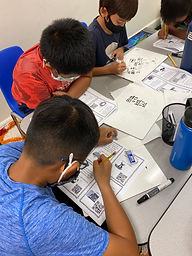## Ms. Kylie

### Target 1​

###### Lesson Type:

Continuation

Data Probability

:

Ratio

Use unit rates to solve problems.

###### 1:

Convert units of measurement using unit rates and proportions.

###### 2:

Use the rules of fractions to solve rates.

###### 3:

Understand that a measurement conversion table identifies unit rates (i.e. 1 in = 2.54 cm).

6th

###### Vocabulary:

Unit Rate, Proportion, Convert

Activities:

-Students used their previous knowledge of unit rates and proportions to convert units of measurement.

-Students were given real world scenarios about world records and used those measurements to convert measurements using unit rates and proportaions.### Home Exploration

###### Guiding Questions:## Absent Students:

### Target 2

:

###### 1:

Define percent increase, decrease, and change.

###### 2:

Recognize that increases and decreases are measures of percent change.

6th

###### Vocabulary:

Percent Increase, Percent Decrease

Activities:

-Students were introduced percent increase and decrease. They were given different scenarios of when there is a percent change and identified if it was a percent increase or decrease.### Home Exploration

###### Guiding Questions:### Target 3

:

###### Vocabulary:

Activities:### Home Exploration